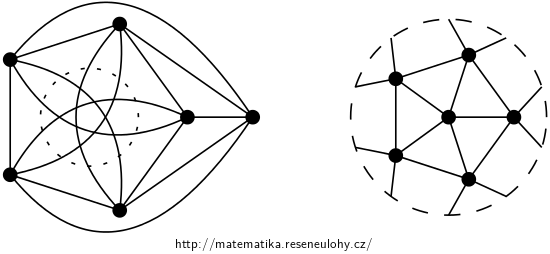## Complete graphs in the projective plane

Which complete graphs $$K_n$$ can be embedded, i.e. drawn without crossing edges, into the projective plane?

• #### Hint

Use Euler’s formula for the projective plane.

Alternatively, use Heawood’s formula.

• #### Solution

Solution via Euler’s formula:

For $$K_n$$: $$| V | (| V | -1) = 2 | E |$$ and $$| F | \leq \frac {2} {3} | E |$$. Euler’s formula for surfaces of higher genus is: $$| V | + | F | = | E | + 2 - g$$. The projective plane has $$g = 1$$. By substituting we get: $\begin {eqnarray*} | V | + | F | & = & | E | + 2 - g \\ | V | + \frac {2} {3} | E | & \geq & | E | + 1 \\ 6 | V | + 4 | E | & \geq & 6 | E | + 6 \\ 6 | V | - 2 | E | & \geq & 6 \\ 6n -n ^ 2 + n & \geq & 6 \\ n ^ 2 -7n + 6 & \leq & 0 \\ (n-6) (n-1) & \leq & 0 \\ n & \leq & 6 \\ \end {eqnarray*}$

Solution via Heawood’s formula:

The projective plane has Euler characteristic $$\chi = 1$$ and the Heawood bound yields $$h (\chi) = \lfloor (7 + \sqrt {49-24}) / 2 \rfloor = 6$$

In both cases, the upper bound is 6, i.e. graphs larger than $$K_6$$ cannot be embedded. You can draw $$K_6$$, for example, starting by the complete graph $$K_5$$ so that it has a cross-cap inside and the outer face has length 5, as in the picture on the left. To the right is the drawing after the circular inversion of the cros-scap.The largest complete graph that can be drawn in the projective plane is $$K_6$$.